# 简单的数学与编程3

6月的时候写了个小demo，有人想问原理或者说怎么实现的，但是一直懒得理。鉴于这个小程序并不是几千行的冗长代码，所以今天简单解释一下吧，希望可以勾起一些人的兴趣。

要讲的demo为一个小球无阻尼运动下与直线碰撞并无能量损失地反弹的小例子。如图：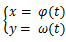（话外音: kao，搞得这么神神叨叨的说白了就一曲线方程嘛）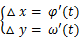Ball:

{

float x, y;   //小球当前相对于屏幕的位置

float dx,dy;  //小球的  y

COLOR  color;  //为便于区分，可以给小球加一个随机的RGB颜色属性

}

k = tanθ = (y1-y0) / (x1-x0)      (θ为直线倾斜角，后面计算反射角要用到)(  Ax + By + C = 0 形式给出如下 )

(y0 – y1)x + (x0 – x1)y + (x0*y1 – x1*y0)
= 0

x1,  C = x0*y1 – x1*y0 )

y = [(y0 – y1)/(x0-x1)]x + [(x0*y1 –
x1*y0) /(x0-x1)]

(x0*y1 – x1*y0) /(x0-x1), B = -1 )

Line:

{

int x0, y0;   //线段端点 P

int x1, y1;   //线段端点 Q

int a, b, c;   // a = A,
b = B, c = C
其中 ABC即为上面所求出的系数

}= 0

距离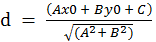k * k’ = -1  è k’ = -1/k

y – y0 = k’(x – x0)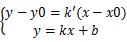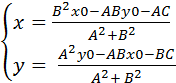PS: 用公式编辑器的感觉真不错，虽然放这里看不出来了）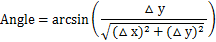(1)     如果△ 0:

①如果△ 0  Angle 本身即为所求

②如果△y < 0,   Angle + 2π 即为所求

(2)     如果△x < 0 π – Angle 即为所求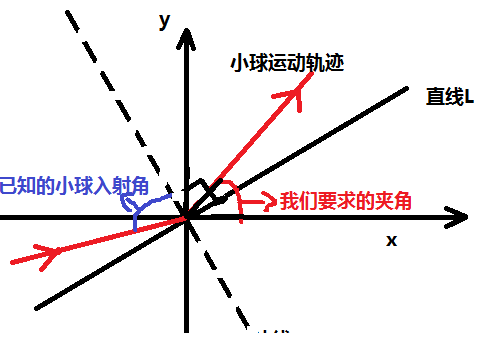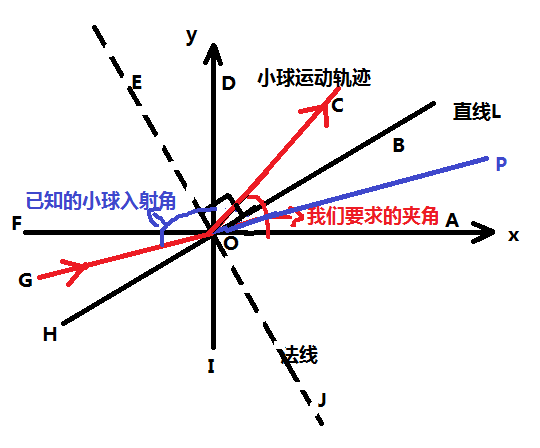GO的延长线 OP 此时 GP为一条直线。此刻我们很清楚地看到，小球的入射方向与x轴的夹角为∠AOP 而∠AOP 就等于我们上一步求得的方向角”Angle”

-A)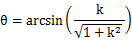如果 0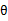本身即为∠AOB

如果k < 0,+ 2π 即为∠AOB

x = speed * cosAOC

y = speed * sinAOC

程序编写结束。

## 3 thoughts on “简单的数学与编程”

1.It抯 awesome for me to have a website, which is useful designed for my know-how. thanks admin wow gold sale http://qaci.eq.edu.au/index.php/member/172769/

2.方教主脑残粉

啦啦啦